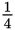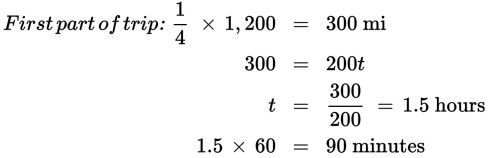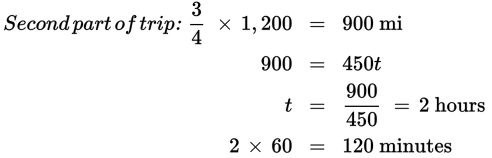# SAT Math Multiple Choice Question 375: Answer and Explanation

### Test Information

Question: 375

15. Three airplanes depart from three different airports at 8:30 AM, all travelling to Chicago O'Hare International Airport (ORD). The distances the planes must travel are recorded in the following table.

From Distance to Chicago (ORD)
Kansas City (MCI) 402
Boston (BOS) 864
Miami (MIA) 1,200

For the firstof the trip, the plane from Miami flew through heavy winds and dense cloud cover at an average speed of 200 mph. For the remaining portion of the trip, the weather was ideal, and the plane flew at an average speed of 450 mph. Due to a backlog of planes at ORD, it was forced to circle overhead in a holding pattern for 25 minutes before landing. At what time did the plane from Miami land in Chicago?

• A. 12:00 PM
• B. 12:25 PM
• C. 12:50 PM
• D. 1:15 PM

Explanation:

B

Difficulty: Hard

Category: Problem Solving and Data Analysis / Rates, Ratios, Proportions, and Percentages

Strategic Advice: Break the question into short steps (first part of trip, second part of trip, circling overhead). Before selecting an answer, make sure you included the results of each step in your calculations.

Getting to the Answer: To get started, you'll need to find the distance for each part of the trip—the question only tells you the total distance. Then, use the formula Distance = rate × time to find how long the plane flew at 200 mph and then how long it flew at 450 mph.This means the plane flew for a total of 90 + 120 = 210 minutes. Next, add the time the plane circled overhead: 210 + 25 = 235 minutes. The total trip took 235 minutes (3 hours and 55 minutes), which means the plane landed at 8:30 + 3 hours = 11:30 + 55 minutes = 12:25 PM.